Download Presentation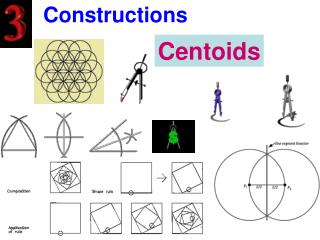Constructions

# Constructions - PowerPoint PPT Presentation

Constructions. Centoids. Review of Prerquisite. To construct a perpendicular bisector you need a . Fish . Let’s begin !. Medians. A Median is a segment connecting the vertex of a triangle to the opposite midpoint.I am the owner, or an agent authorized to act on behalf of the owner, of the copyrighted work described.
Download Presentation## Constructions

An Image/Link below is provided (as is) to download presentation

Download Policy: Content on the Website is provided to you AS IS for your information and personal use and may not be sold / licensed / shared on other websites without getting consent from its author.While downloading, if for some reason you are not able to download a presentation, the publisher may have deleted the file from their server.

- - - - - - - - - - - - - - - - - - - - - - - - - - E N D - - - - - - - - - - - - - - - - - - - - - - - - - -
Presentation Transcript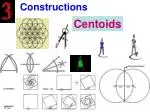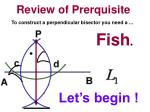Review of Prerquisite

To construct a perpendicular bisector you need a ...

Fish.

Let’s begin !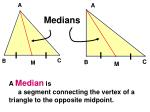Medians

A Median is

a segment connecting the vertex of a triangle to the opposite midpoint.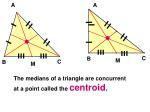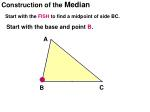Construction of the Median

Start with the FISH to find a midpoint of side BC.

Start with the base and point B.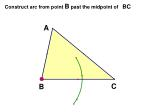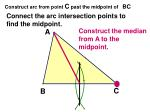Construct arc from point C past the midpoint of BC

Connect the arc intersection points to find the midpoint.

Construct the median from A to the midpoint.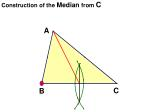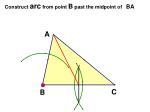Construct arc from point A past the midpoint of BA

Connect the arc intersection points to find the

midpoint.

Construct the median from C to the midpoint.Two intersecting lines determine a point.

CentroidIt is only necessary to draw 2 medians.

The third median would only intersect the other lines at the same point.

We will now look at several examples of centroids to solidify your understanding.1

3

2

4Construction of the Median

Start with the FISH to find a midpoint of side BC.

Start with the base and point B.Construct arc from point C past the midpoint of BC

Connect the arc intersection points to find the midpoint.

Construct the median from A to the midpoint.Construct arc from point A past the midpoint of BA

Connect the arc intersection points to find the

midpoint.

Construct the median from C to the midpoint.Two intersecting lines determine a point.

CentroidWhen two medians intersect then they divide each other into a small segment and a large segment.

Let’s look at several situations.The ratio is always 2:1

Therefore…

10

If DF = 5, then AD = _____

?

510

If DF = 5, then AD = _____

?

76

If AD = 12, then DF = _____

12

?8

If AD = 16, then DF = _____

16

?Summary

1. A Median is

a segment connecting the vertex of a triangle to the opposite midpoint.

2. The three medians of a triangle are concurrent.

3. The point of concurrency is called

a centroid.Summary

4. When two medians intersect then they divide each other into a large segment and a small segment.Summary

5. The centroid is always inside the triangle.

6. To construct the median you…

You construct a fish on 2 sides.

You connect the opposite vertex to the midpoint.C’est fini.

Good day and good luck.

That’s all folks.

A Senior Citizen Production